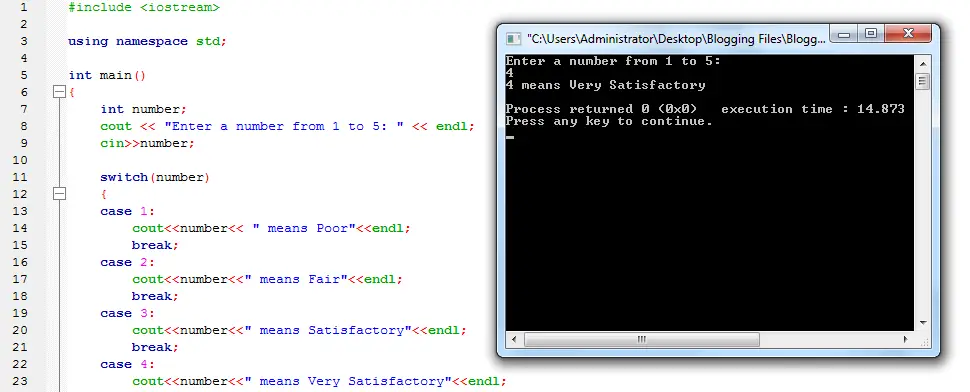# Switch Statement in C++• Version
• File Size 1.40 KB
• File Count 1
• Create Date May 9, 2016
• Last Updated May 9, 2016

# Switch Statement in C++

Switch Statement in C++

A program in c++ that showcase the use and function of a switch statement. Switch statement is just like if elseif statement, what it does is to execute a statement that matches the expression’s value. If it has no match then the default case will be executed.

Source code:

```#include < iostream >

using namespace std;

int main()
{
int number;
cout << "Enter a number from 1 to 5: " << endl;
cin >> number;

switch(number)
{
case 1:
cout << number << " means Poor"  << endl;
break;
case 2:
cout << number <<" means Fair" << endl;
break;
case 3:
cou t<< number  << " means Satisfactory" << endl;
break;
case 4:
cout << number << " means Very Satisfactory" << endl;
break;
case 5:
cout << number << " means Outstanding" << endl;
break;
default:
cout << number << " is invalid" << endl;
break;
}

return 0;
}
```